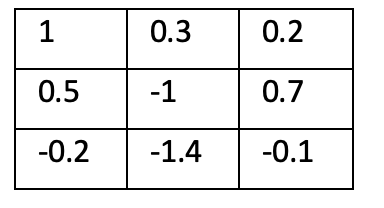# Normalized convolution layer

I would like to create a custom convolution layer in which, after each back-propagation and the weight’s update, I need to transfer the weight of my kernel matrix to a specific format: the central value must be -1, and the sum of the rest must be 1.This might work.

``````import torch
import torch.nn.functional as F

#define images and conv2d output channels
images=torch.rand((5, 3, 16, 16))
out_channels=3

#define custom kernel
kernel=torch.tensor([[[[0, 1, 0], [1, -1, 1], [0, 1, 0]]]]).float()

#repeat kernel for correct input channels and output channels
kernel=torch.cat([kernel]*images.size(), dim=1)
kernel=torch.cat([kernel]*out_channels, dim=0)

#pass through conv2d
``````

But the above won’t involve updates. This would be how to apply a kernel in conv2d. You would need to write some function to update it according to your requirements.

In this case, you are referring to the kernel weight initialization. These constraints should be applied during training. Below is an implementation that I am not certain is correct; if anyone has any comments, please let me know.

``````class Constlayer(nn.Module):
"""
doc
"""
def __init__(self, numch: int=128, centralval=1.0):
super().__init__()
self.register_parameter(name="const_weight", param=None)
self.numch = numch
self.centralval = centralval
self.const_weight = nn.Parameter(torch.randn(size=[numch, 1, 5, 5]), requires_grad=True)

def normalize(self):